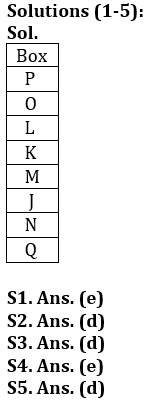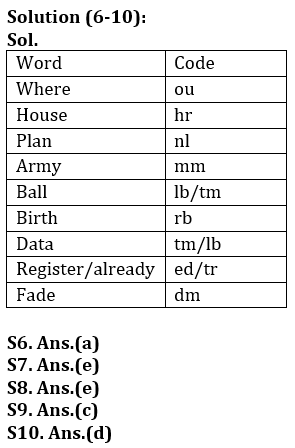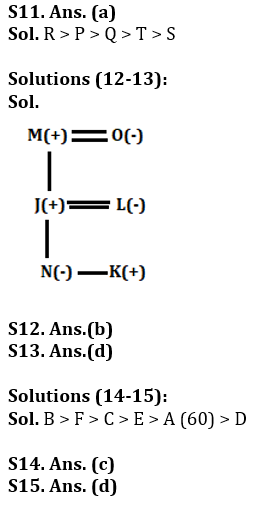Latest Banking jobs   »

# Reasoning Quiz For Bank of Baroda AO 2023-10th March

Directions (1-5): Study the information carefully and answer the questions given below.
There are eight boxes J, K, L, M, N, O, P and Q are placed one above another. Two boxes are placed between Box K and Box N. Box Q is placed immediately below Box N. One box is placed between Box P and Box L. Box M is placed one of the above places of N and one of the below places of Box O but not immediate above or below. Box J and Box O is not placed above Box P.

Q1. Which of the following box is placed just below box L?
(a) N
(b) Q
(c) M
(d) O
(e) None of these

Q2. Which of the following box is placed just above box N?
(a) Q
(b) L
(c) M
(d) J
(e) None of these

Q3. How many boxes are placed between box O and box N?
(a) Three
(b) Two
(c) One
(d) Four
(e) None

Q4. What is the position of box M?
(a) Third from the top
(b) Second from the top
(c) Third from the bottom
(d) Second from the bottom
(e) None of these

Q5. Four of the following five are alike in certain way based from a group, find the one that does not belong to that group?
(a) P-L
(b) O-K
(c) N-M
(d) L-N
(e) Q-J

Directions (6-10): Study the following information carefully and answer the questions given below:
In a certain code language
‘Where house plan army’ is written as ‘nl am hr ou’,
‘Ball house birth data’ is written as ‘tm rb lb hr’,
‘Army plan register already’ is written as ‘ed nl am tr’,
‘Fade plan data ball’ is written as ‘tm lb nl dm’.

Q6. What is the code for ‘Fade’?
(a) dm
(b) nl
(c) lb
(d) tm
(e) None of these

Q7. Which of the following may be the code for ‘already house plan’?
(a) ed hr rb
(b) tr nl hr
(c) rb hr tr
(d) ed nl hr
(e) Either (b) or (d)

Q8. What is the code for ‘Register’?
(a) tr
(b) am
(c) nl
(d) ed
(e) Can’t be determined

Q9. What does ‘rb’ stand for?
(a) House
(b) Ball
(c) Birth
(d) Data
(e) None of these

Q10. What is the code for ‘Plan’?
(a) am
(b) hr
(c) lb
(d) nl
(e) Can’t be determined

Q11. There are five persons i.e., P, Q, R, S and T. If S is shorter than T and P. Q is taller than T who is not the taller than P. Q is taller than only two persons. P is not the tallest, then who is the second tallest person among all?
(a) P
(b) S
(c) Q
(d) R
(e) None of these

Directions (12-13): Study the information carefully and answer the questions given below.
J, K, L, M, N and O are six members of a family. There are equal numbers of male and female in the family. J is the son of M and N is the daughter of J. K is the child of L who is the mother of N. O is paternal grandmother of K.

Q12.How is O related to L?
(a) Daughter-in-law
(b) Mother-in-law
(c) Mother
(d) Father-in-law
(e) Father

Q13.How is K related to M?
(a) Daughter
(b) Father
(c) Son
(d) Grandson
(e) Granddaughter

Directions (14-15): Study the following information carefully and answer the given questions.
Amongst Six friends A, B, C, D, E and F, each got different marks in the examination. E score more than A. C score less than F. More than three persons score less than B. E does not score more than C. A does not score lowest marks and F does not score highest marks. The one who scores second lowest score 60 marks.

Q14. Who among the following person score fourth highest?
(a) C
(b) A
(c) E
(d) D
(e) None of these

Q15. If C score 80 marks, then what may be the score of E?
(a) 63 marks
(b) 70 marks
(c) 52 marks
(d) Either (a) or (b)
(e) Can’t be determined

Solutions## FAQs

### What is the selection process of Bank of Baroda AO 2023?

The selection process of Bank of Baroda AO consists of Online exam and Interview

#### Congratulations!Union Budget 2023-24: Free PDF